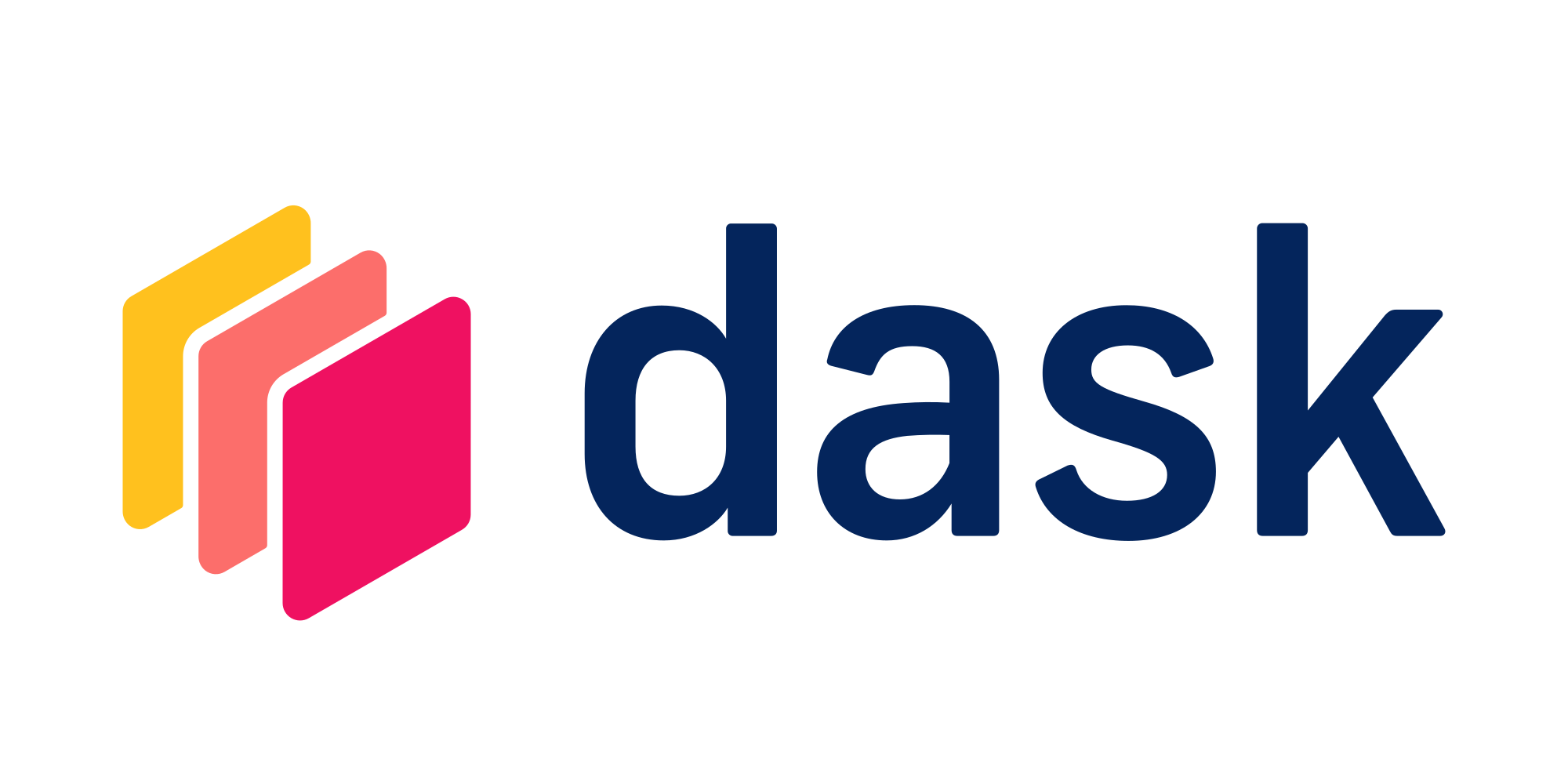Use Dask Futures as a drop in replacement for Python built in concurrencyTry this example in seconds on Saturn Cloud

Dask Futures allows you to use the syntax of the standard Python `concurrent.futures` library but to have the computations run across a distributed cluster of machines. It’s an alternative to Dask Delayed which has its own particular syntax and lazy evaluation–here everything mimics the built in Python methods.

This example will show how to have Python code run in parallel using Dask Futures. This will use a Formula One lap time dataset from kaggle. We will be finding a range of lap times for a formula one driver. First we create a pandas DataFrame out of csv file and filter out data for a particular driver. We create three functions: finding maximum lap time, finding minimum lap time and then getting the range out of these two functions.

``````from dask_saturn import SaturnCluster

client = Client(SaturnCluster())
``````

After running the above command, it’s recommended that you check on the Saturn Cloud resource page that the Dask cluster as fully online before continuing. Alternatively, you can use the command `client.wait_for_workers(3)` to halt the notebook execution until all three of the workers are ready.

Next, we load the data, filter it, and define the functions:

``````import pandas as pd

)

d_20 = f1[f1["driverId"] == 20]

def max_lap(x):
return max(x)

def min_lap(x):
return min(x)

def range_lap(a, b):
return a - b
``````

Here is how to compute the range of lap times using pandas:

``````a = max_lap(d_20.milliseconds)
b = min_lap(d_20.milliseconds)
c = range_lap(a, b)

c
``````

Now let us add scalability to code above using Futures. We have 3 tasks below , finding the maximum laptime, minimum laptime and range. We have used future’s submit method, to submit each task individually. `client.submit` allows us to submit data or tasks directly to the cluster. This method returns a future object and the results stay in remote thread. To convert Future into a concrete value you call result method as shown in last line.

``````a = client.submit(max_lap, d_20.milliseconds)
b = client.submit(min_lap, d_20.milliseconds)
c = client.submit(range_lap, a, b)
c.result()
``````

This computation was done concurrently on the Dask cluster and only required a small change of adding `client.submit` when calling the functions. While this was a simple example, with larger data and more complex functions it can be very useful.

For more details on using Dask Futures, see the official Dask Futures Documentation.

``````from dask_saturn import SaturnCluster

client = Client(SaturnCluster())

import pandas as pd

)

d_20 = f1[f1["driverId"] == 20]

def max_lap(x):
return max(x)

def min_lap(x):
return min(x)

def range_lap(a, b):
return a - b

a = max_lap(d_20.milliseconds)
b = min_lap(d_20.milliseconds)
c = range_lap(a, b)

c

a = client.submit(max_lap, d_20.milliseconds)
b = client.submit(min_lap, d_20.milliseconds)
c = client.submit(range_lap, a, b)
c.result()

``````